﻿

##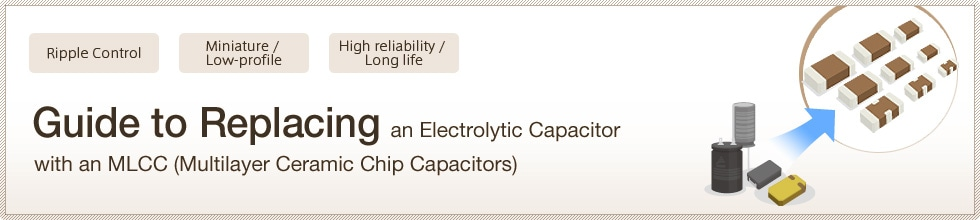### Guide to Replacing an Electrolytic Capacitor with an MLCC Overview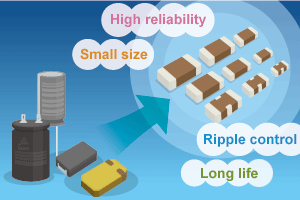Summary Guide to Replacing Electrolytic Capacitors with MLCCs

### Why are electrolytic capacitors now being replaced with MLCCs?

###### Electrolytic Capacitor Replacement is Possible Today due to Large Capacitance in MLCCs

Figure 1: The frequency band used by various capacitors and the
range of capacitance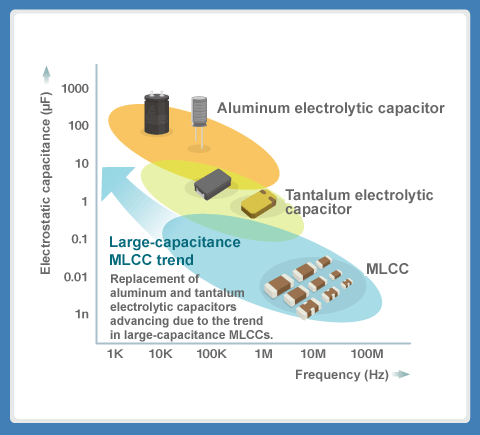Along with the increasing high integration of the primary LSI and IC components in electronic devices, there has been a trend toward low voltage in the power supplies which supply these components. In addition, power consumption has also increased with the progression of multi-functionality and the trend toward the use of high current continues. To support the trends toward low voltage and high current, the power supplies of electronic devices have switched from intermediate bus converters to adopt dispersed power supply systems which place multiple miniature DC-DC converters (POL converters) near the LSI and IC loads.

In a POL converter, multiple capacitors are externally attached. Previously, aluminum and tantalum capacitors were used in particular due to the need for large capacitance in output smoothing capacitors.
However, the difficulty in miniaturizing these electrolytic capacitors is a hindrance to reducing the circuit space. In addition, they possess significant problems with self-heating due to ripple currents.

The MLCCs used in many electronic devices are capacitors with superior characteristics, but their capacitance is comparatively low and they have been used primarily in filter and high-frequency circuits. However, with the advancements in the technology for thinning and multi-layering the MLCC dielectric materials in recent years, large-capacitance MLCCs that are between several dozen to over 100 μF have been developed, enabling the replacement of electrolytic capacitors.

###### Precautions When Using Various Capacitors

The primary features and cautions on usage of MLCC, aluminum electrolytic capacitors and tantalum electrolytic capacitors are indicated below. It is important to understand these cautions on usage as well as the merits and demerits of these capacitors when replacing them with an MLCC.
While large-capacitance MLCCs make it possible to replace electrolytic capacitors, it is important to note their shortcoming which is the large rate of change in capacitance due to temperature and DC bias. In addition, an ESR which is too low has adverse effects and may lead to anomalous oscillations in power supply circuits.
» Question note: Why do anomalous oscillations occur when an MLCC is used as an output capacitor for a DC-DC converter?
» Question note: What is the phase compensation that is used to prevent anomalous oscillations?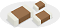MLCC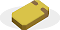Tantalum
electrolytic capacitor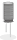Aluminum
electrolytic capacitor
Primary features
• Miniature, low-profile
• Low ESR (equivalent series resistance)
• No polarity
• Large-capacitance
• Superior DC bias characteristics
• Large-capacitance
• Inexpensive
Cautions on usage
• Large change in capacitance due to temperature and DC bias (direct current applied)
• Low ESR is an advantage, but may also cause anomalous oscillations in power supply circuits
• Comparatively high ESR, significant self-heating due to ripple currents
• Low rated voltage
• Large form factor
• Short lifetime in high-temperature environments
• High ESR, significant self-heating due to ripple currents
###### Large-Capacitance Electrolytic Capacitors Which Tend to Have a Short Lifetime Due to Significant Self-Heating

Figure 2: Comparative example of capacitor's self-heating due to ripple
currents (frequency: 100 kHz)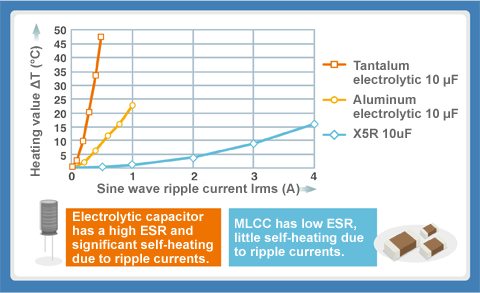The capacitor's ESR changes according to the frequency.
With the capacitor ESR at a certain frequency set as "R" and the ripple current set as "I", "RI2" becomes the power heat loss and the capacitor self-heats.

While large-capacitance is acquired using an electrolytic capacitor, significant heat develops due to ripple current and a high ESR, which is a weakness of electrolytic capacitors.

The upper limit of the ripple current which the capacitor allows is called the "allowable ripple current". The life of the capacitor will decrease when the usage exceeds the allowable ripple current.

##### Note: ESR and ripple currents

Figure 3: ESR (equivalent series resistance)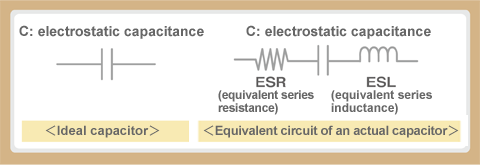The ideal capacitor would possess only the properties of capacitance, but in reality it also contains resistor and inductor components due to the electrodes. The resistor component, not shown in the ideal capacitor, is called the "ESR (equivalent series resistance)" and the inductor component is called the "ESL (equivalent series inductance)".

Figure 4: Ripple currents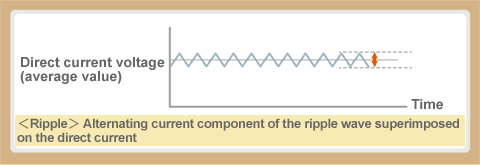DC (Direct Current) is when current flows in one direction, but in dc power supplies in addition to DC current there are various superimposed alternating current components which adds ripple to the current. For example, the direct current resulting from the rectification (full-wave rectification) of commercial alternating current contains pulsating ripple currents at twice the cycle of the commercial alternating current. In addition, the pulsating current of the switching cycle in a switching DC-DC converter is superimposed on the direct current voltage. This is called the "ripple current".

###### Aluminum Capacitors Have a Lifetime of 10 Years

Aluminum electrolytic capacitors are widely used in electronic devices, because they have high capacitance and are inexpensive, but caution is required due to their limited lifetime. The typical lifetime of an aluminum electrolytic capacitor is said to be ten years. This is because the capacitance decreases as the electrolytic solution dries up (capacitance loss).

The amount of electrolytic solution lost is related to temperature and closely follows the "Arrhenius equation" of chemical reaction kinetics. If the usage temperature increases by 10°C, then the lifetime will be reduced to half. If the usage temperature decreases by 10°C, then the lifetime will be doubled, so it also called the "10°C double" rule. For this reason, the lifetime is reduced even more when used under conditions with significant self-heating due to ripple currents.

The drying up of the electrolytic solution also increases the ESR. Attention should be noted that the peak value of the ripple voltage does not exceed the rated voltage (withstanding voltage) when the ripple voltage is superimposed on the direct current voltage. A capacitor used in a power supply circuit has a rated voltage that is three times of the input voltage.

Figure 5: The rated voltage range of various capacitors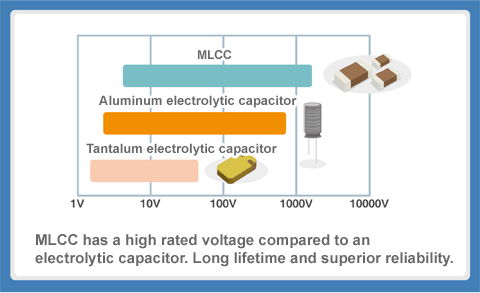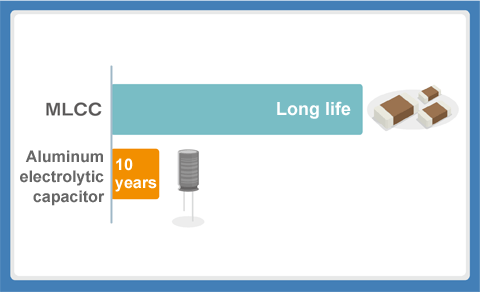### MLCC Replacement Example: Step-down DC-DC Converter

###### Replacing the Output Capacitor in a Step-down DC-DC Converter

Capacitor heat generation due to ESR and ripple currents is a predominant issue in the output capacitors of power supply circuits.
Figure 7 shows the fundamental circuit of a miniature step-down DC-DC converter which is used as a POL converter in many electronic devices.

This type of output capacitor is the primary target for replacement of electrolytic capacitors with MLCCs in DC-DC converters as a solution for the self-heating issue, space reduction and improved reliability.

Figure 7: Fundamental circuit of a POL converter
(step-down DC-DC converter)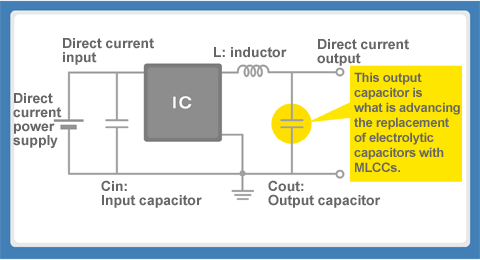##### Note: Fundamental circuit of a POL converter (step-down DC-DC converter)

Figure 8 shows the fundamental circuit of the miniature step-down DC-DC converter which is used as a POL converter in many electronic devices.
The main converter circuit has been made into an IC, and the capacitor and inductor are attached externally on the PCB (internally attached products also exist).
The capacitor which comes before the IC is called the "input capacitor (Cin)" and the one that comes after is the "output capacitor (Cout)". In addition to collecting an electrical charge and smoothing the output voltage, the output capacitor in a DC-DC converter plays the role of grounding and removing the ripple component of the alternating current.

###### Comparison of Output Capacitor Characteristics in a Step-down DC-DC Converter

The output voltages of the step-down DC-DC converter's output capacitors were compared using the following type of evaluation board. The capacitors compared were a typical aluminum electrolytic capacitor, a tantalum electrolytic capacitor, a functional polymer aluminum electrolytic capacitor and an MLCC with a capacitance of 22μF.

Figure 8: Comparative verification of the output voltage of various electrolytic capacitors with an MLCC (22μF products)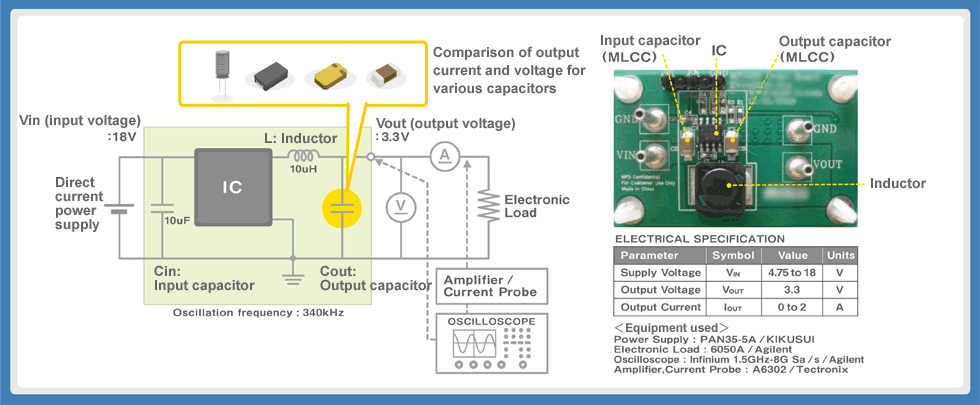###### MLCC Has Small Ripple Currents and Little Self-Heating Due to the Low ESR

Based on the previously stated conditions, a comparison was made of the output current and output voltage of the typical aluminum electrolytic capacitor, a tantalum electrolytic capacitor, a functional polymer aluminum electrolytic capacitor and an MLCC with a capacitance of 22μF.
The ESR in descending order of size is the typical aluminum electrolytic capacitor > tantalum electrolytic capacitor > functional polymer aluminum electrolytic capacitor > MLCC. The ripple voltage, which causes the self-heating, follows a similar pattern. The functional polymer aluminum electrolytic capacitor uses a conductive polymer as the electrolyte and is a type designed for a low ESR. Compared to the typical aluminum electrolytic capacitor, the ripple voltage is significantly smaller, but the form factor is slightly large and the price is expensive.

Figure 9: Output behavior test results (22μF products) of various types of electrolytic capacitors with an MLCC (B characteristic)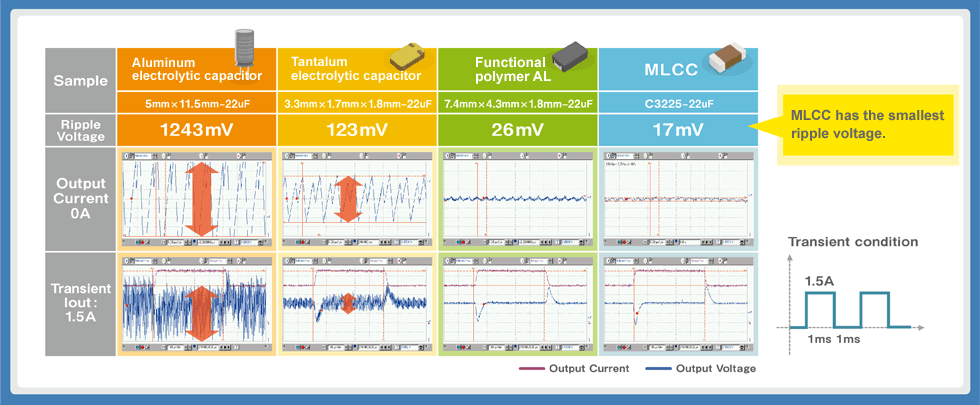The impedance-frequency characteristics and ESR-frequency characteristics for each are as follows.

Figure 10: The impedance-frequency characteristics and ESR-frequency characteristics for various capacitors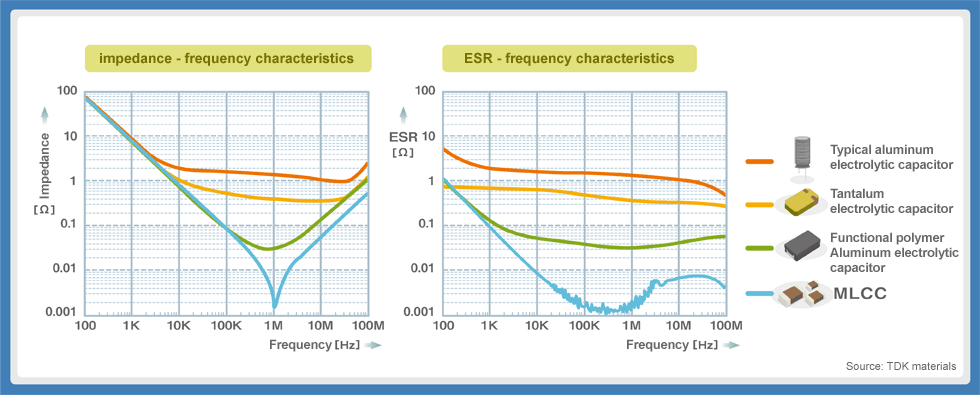As the capacitor ESR becomes lower, the ripple voltage can be kept to a smaller amount. As shown in the graph below, the ESR of the MLCC is about several mmΩ, which is extremely small. For this reason, the MLCC displays optimal performance as a replacement for an electrolytic capacitor.

Figure 11: The relationship between ESR and the ripple voltage (switching frequency of 340 kHz)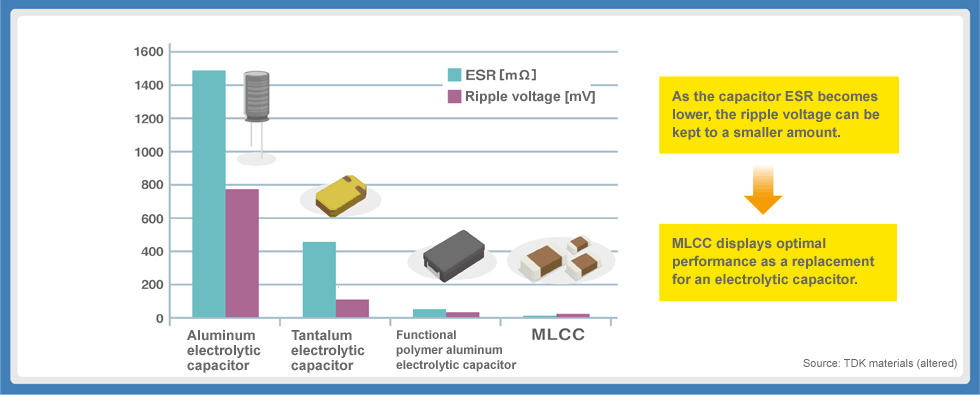###### Merits of Replacing an Electrolytic Capacitor in a DC-DC Converter with an MLCC

Replacing an electrolytic capacitor with an MLCC provides various advantages such as ripple control as well as space reduction of the circuit board due to the miniature and low-profile form factor, a long lifetime and an improvement in reliability.

#### Ripple Control, High Reliability, Long Lifetime

Self-heating due to ripple currents in capacitors with a high ESR shortens the lifetime of the capacitor.
The ESR of an MLCC is lower than that of an electrolytic capacitor by double-digits and the long lifetime improves the reliability.

Figure 12: Ripple control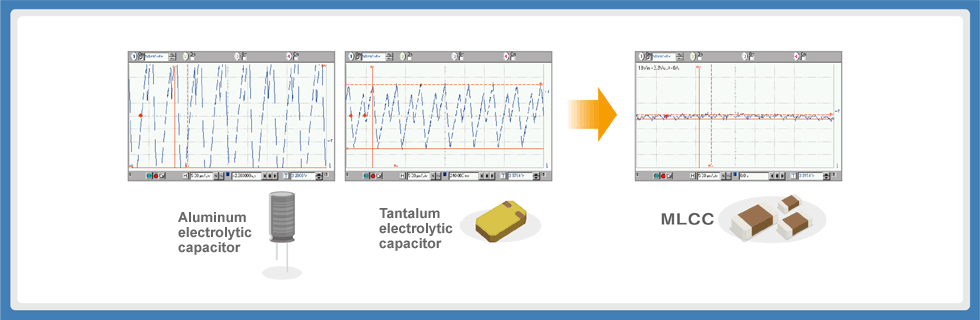#### Miniaturization

The switch to miniature, low-profile MLCCs enables the circuit board space to be reduced.

Figure 13: Switching from an aluminum electrolytic capacitor to an MLCC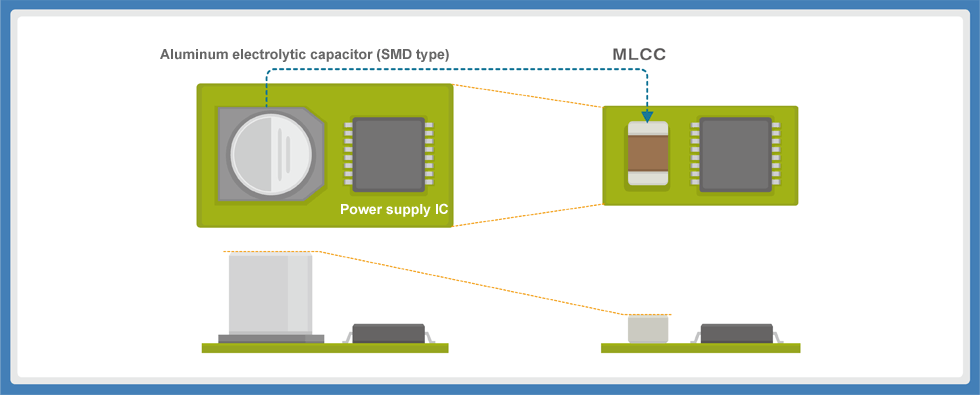##### Question note: Can the ripple voltage be controlled by increasing the capacitance of the electrolytic capacitor?

The ESR of an electrolytic capacitor decreases slightly when the capacitance increases. However, it is fundamentally difficult to control the ripples by increasing the capacitance. This is because the time constant increases together with the increase in capacitance.
The response speed of a transient phenomenon such as the charging and discharging process of a capacitor can be expressed as the time constant index called (T). In an RC circuit consisting of the resistance (R) and the capacitor (C), the time constant becomes T=RC (R is in units of ohms [Ω], the capacitance C is in units of farads [F]). The time required for charging and discharging of the capacitor is short when the time constant is small and becomes longer as the time constant increases.
The time constant becomes extremely large when using an electrolytic capacitor with an excessively large capacitance. In a DC-DC converter with repeated switching of a short duration, the discharging does not complete within the switch OFF time and charge remains in the electrolytic capacitor. As a result, the voltage does not sufficiently decrease, distortions occur in the voltage waveform, and the output becomes unstable, which does not allow for favorable ripple control (Figure 14).

Figure 14: Distortions occurring in the waveform of a large capacitance aluminum electrolytic capacitor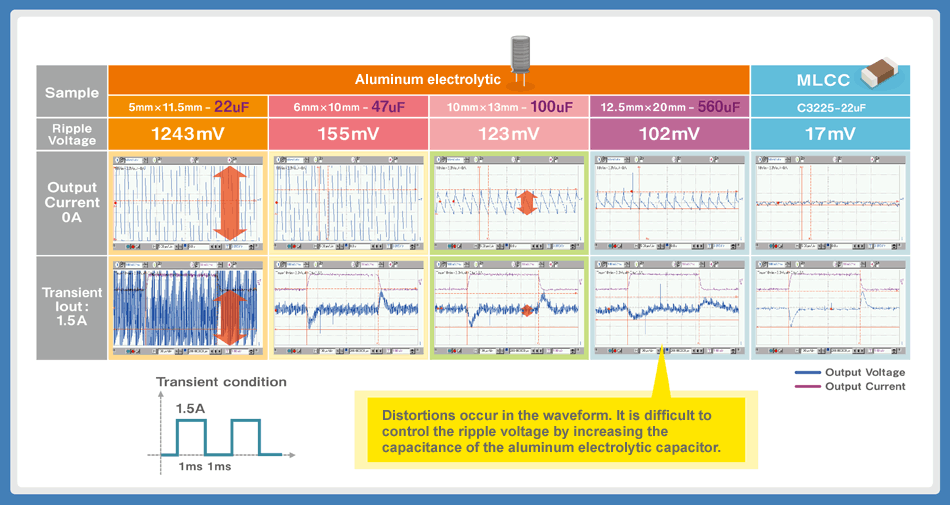MLCCs, on the other hand, do not have this kind of problem because of the low ESR over a wide frequency band, which achieves favorable ripple control in the place of an electrolytic capacitor.

Figure 15: The impedance and ESR of an electrolytic capacitor
and an MLCC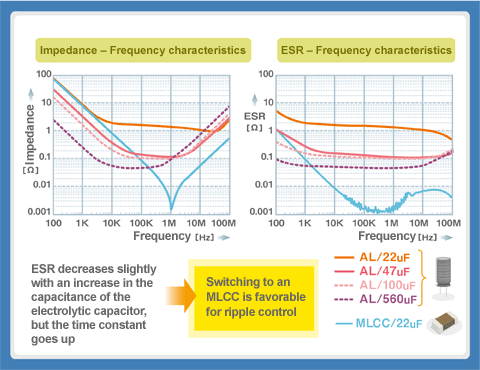##### Question note: Why do anomalous oscillations occur when an MLCC is used as the output capacitor in a DC-DC converter?

The low ESR is a feature of the MLCC, but it is so much lower compared to an aluminum electrolytic capacitor that on the contrary, the output voltage of the DC-DC converter becomes unstable and causes oscillations to occur.
As shown in the figure on the right, the DC-DC converter compares the output voltage with the reference voltage, amplifies the error amount with the error amp (error amplifier), and performs negative feedback to achieve a constant and stable direct current voltage. However, signal phase lag occurs due to the inductor (L) and the capacitor (C) of the smoothing circuit. As the phase lag approaches 180°, a positive feedback condition is created, causing it to become unstable and oscillate.

Figure 16: Negative feedback circuit in a DC-DC converter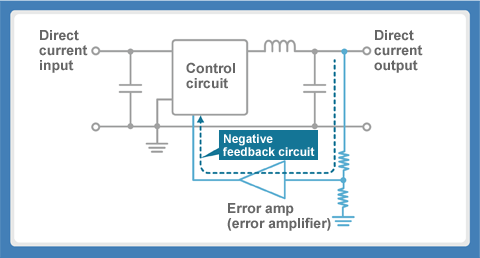##### Question note: What is the phase compensation that is used to prevent anomalous oscillations?

There is a board diagram used as a chart to determine whether the negative feedback will operate in a stable manner. The horizontal axis of the graph is the frequency and the vertical axis is the gain and phase.
As the phase lag due to the inductor (L) and capacitor (C) approaches 180°, it creates positive feedback and the output becomes unstable. However, setting the gain to 1 or less (0 dB or less) even when the phase lag is 180° converges the signal and can prevent the oscillations.
Connect a capacitor and resistor near the error amp to reduce the phase lag and adjust to cancel it. This is called "phase compensation". Previous designs which used an aluminum electrolytic capacitor with a high ESR for the output capacitor did not have this problem. However, the MLCC has insufficient compensation, which causes anomalous oscillations, so caution is required when replacing capacitors.

Figure 17: Board diagram (gain and phase/frequency characteristics)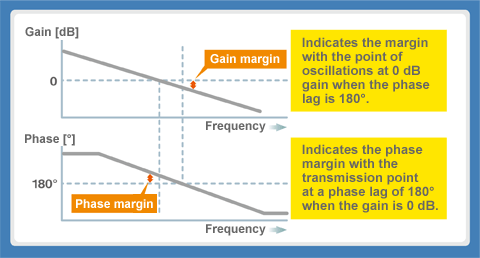Figure 18: Phase compensation circuit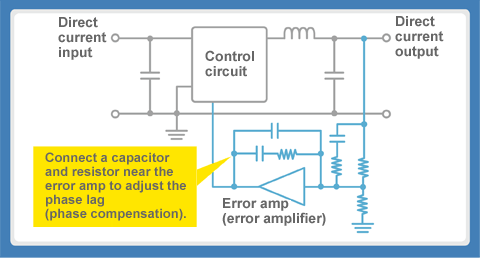### MLCC Replacement Example: Decoupling Capacitor (Bypass Capacitor)

###### Replacing a Decoupling Capacitor (Bypass Capacitor)

Previously, electrolytic capacitors and MLCCs where connected in parallel for decoupling in an analog circuit, but with the productization of large-capacitance MLCCs, the replacement of electrolytic capacitors with MLCCs is advancing.

In particular, large-capacitance is required to reduce impedance due to the large ESR in an aluminum electrolytic capacitor. However, an MLCC does not need the same capacitance as an aluminum electrolytic capacitor, because low ESR is a feature of the MLCC. The miniaturization and low-profile of the MLCC also achieves a reduction in circuit board space, and the long lifetime and superior reliability are also replacement advantages.

Figure 19: POL converter (step-down DC-DC converter)
fundamental circuit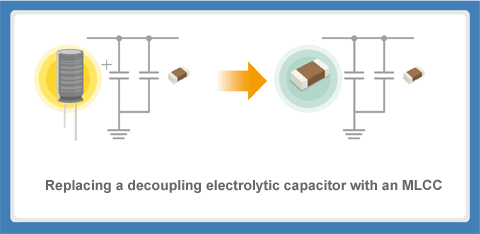##### Note: Decoupling capacitor

When a capacitor is connected in parallel to the power supply line of an IC, there is impedance in the power supply line, which is not shown in the circuit diagram, which can change the power supply voltage and cause a malfunction or interference between circuits.

A capacitor is connected in parallel to control the voltage variations through charging and discharging. Additionally, since a capacitor passes alternating current, it removes or directs ripple noise to ground. This is called a "decoupling capacitor" (also called a "bypass capacitor").

Figure 20: The role of a decoupling capacitor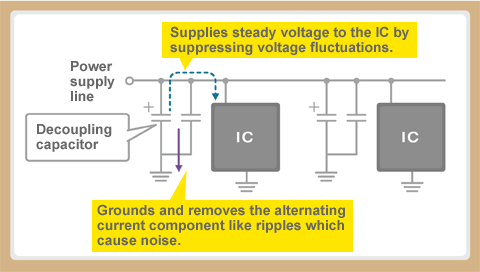For decoupling use, the ideal capacitor would have low impedance across a wide band of frequencies from low to high, but in reality the impedance-frequency characteristics of a capacitor follow a V-shaped curve.

The frequency at the trough of the V-shape is called the "self-resonating frequency" (SRF), and it functions as a capacitor in the region below the SRF. For this reason, capacitors with different characteristics are typically connected in parallel to cover a wide frequency band in decoupling applications.

Figure 21: The role of the decoupling capacitor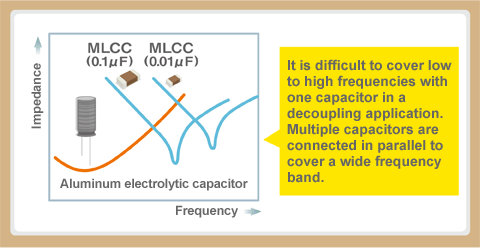##### Question note: What is the anti-resonance phenomenon that occurs when an MLCC is used as a decoupling capacitor?

The low ESR is a feature of the MLCC, but this can have adverse effects even in decoupling applications. For example, multiple MLCCs are connected in parallel for decoupling in an IC operating with a large current and a low voltage. The capacitor functions as a capacitor below the SRF (self-resonating frequency) frequency band and as an inductor above the SRF.

For this reason, when the SRFs of two MLCCs are close to each other, an LC parallel resonance circuit is created between the SRFs by an inductor and a capacitor and it is easy for them to oscillate. This phenomenon is called "anti-resonance". Anti-resonance creates intense impedance peaks which weaken the noise removal effect at that frequency. This can cause the power supply voltage to become unstable and the circuit to malfunction.

Figure 22: Parallel MLCC connections for decoupling and the
anti-resonance problem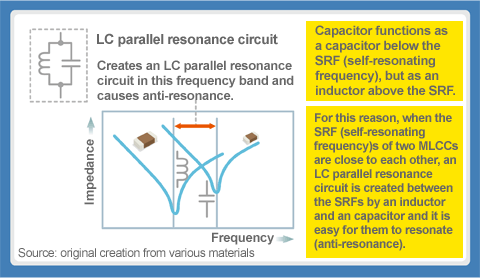### Guide to Replacing an Electrolytic Capacitor with an MLCC

This section explains how to select the optimal MLCC for your intended application when replacing an electrolytic capacitor with an MLCC. Please use it to improve the reliability of your products.

#### Caution: Capacitance in materials with a high-dielectric constant will change according to the applied voltage

The MLCC is a superior capacitor, but it also has weaknesses. The capacitance of an MLCC changes according to the applied voltage. This is called the "DC bias characteristic" when a DC voltage is applied. Changes in the capacitance (dependent on the DC bias) are rarely seen with a low-dielectric constant MLCC (type 1) but do appear with a high-dielectric constant MLCC (type 2).

This is caused by the intrinsic polarization of the ferroelectric (BaTiO3, etc.) used by the high-dielectric constant material. For this reason, please consider the dielectric characteristics, the voltage used and the withstanding voltage when making your selection if it is to be used when applying a DC voltage. There is also a tendency for the capacitance to be significantly reduced in miniature-sized capacitors. The DC bias characteristics must also be considered when selecting the capacitance.

Figure 23: Rate of change in the capacitance
- DC bias characteristic example (high-dielectric constant)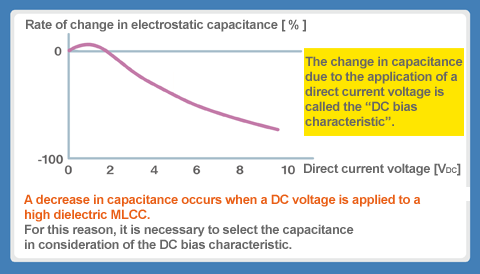Figure 24: Effects of the DC bias characteristic (comparison of effective capacitance when 3.3V is applied)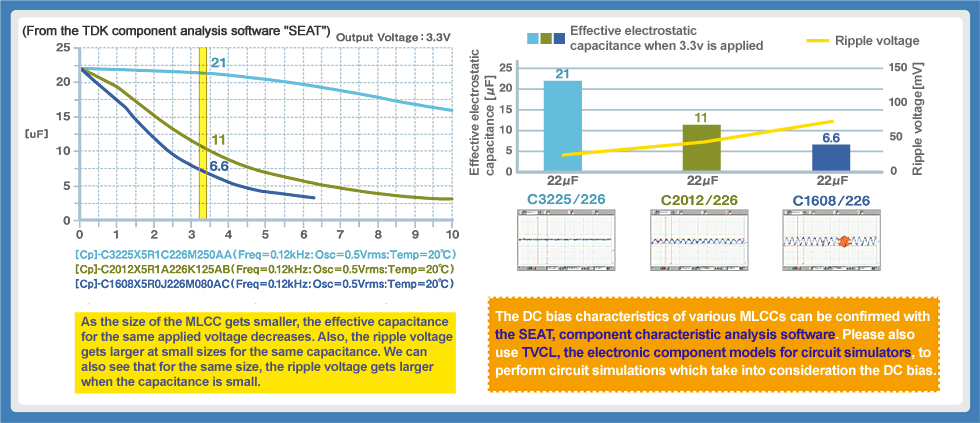###### Optimal MLCC Lineup for Replacing Electrolytic Capacitors

By clicking on the various parameters below for an existing replacement capacitor, you can see the recommended MLCC product.
* Note that information provided here does not guarantee product compatibility.

How to select the optimal MLCC to replace an electrolytic capacitor (PDF)

TDK offers an abundant lineup of MLCCs for achieving success in the replacement of aluminum and tantalum electrolytic capacitors. Please select the right MLCC for your application to improve the reliability of your products.

### Summary Guide to Replacing an Electrolytic Capacitor with an MLCC

• In recent years, the productization of high-capacitance MLCCs of several dozen to over 100 µF has made it possible to replace tantalum and aluminum electrolytic capacitors.
• The switch to MLCCs in a wide range of consumer and industrial devices is advancing due to their high rated voltage, superior ripple control, long lifetime and high reliability.

*The weakness of the high-dielectric MLCC is the reduction in capacitance due to temperature or the application of a DC voltage (temperature characteristic, DC bias characteristic). In addition, the extremely low ESR feature can cause anomalous oscillations and anti-resonance to occur, so caution is needed when replacing capacitors.